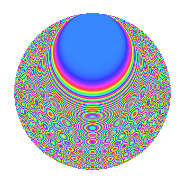# Properties

 Label 1512.2.bkLevel 1512 Weight 2 Character orbit bk Rep. character $$\chi_{1512}(1027,\cdot)$$ Character field $$\Q(\zeta_{6})$$ Dimension 256 Sturm bound 576

# Related objects

## Defining parameters

 Level: $$N$$ = $$1512 = 2^{3} \cdot 3^{3} \cdot 7$$ Weight: $$k$$ = $$2$$ Character orbit: $$[\chi]$$ = 1512.bk (of order $$6$$ and degree $$2$$) Character conductor: $$\operatorname{cond}(\chi)$$ = $$56$$ Character field: $$\Q(\zeta_{6})$$ Sturm bound: $$576$$

## Dimensions

The following table gives the dimensions of various subspaces of $$M_{2}(1512, [\chi])$$.

Total New Old
Modular forms 600 256 344
Cusp forms 552 256 296
Eisenstein series 48 0 48

## Trace form

 $$256q + O(q^{10})$$ $$256q - 16q^{16} - 20q^{22} - 128q^{25} + 10q^{28} + 30q^{40} + 6q^{46} - 8q^{49} + 18q^{52} + 4q^{58} - 60q^{64} - 64q^{67} - 102q^{70} + 24q^{73} + 66q^{82} + 40q^{88} - 24q^{91} + 18q^{94} + O(q^{100})$$

## Decomposition of $$S_{2}^{\mathrm{new}}(1512, [\chi])$$ into newform subspaces

The newforms in this space have not yet been added to the LMFDB.

## Decomposition of $$S_{2}^{\mathrm{old}}(1512, [\chi])$$ into lower level spaces

$$S_{2}^{\mathrm{old}}(1512, [\chi]) \cong$$ $$S_{2}^{\mathrm{new}}(56, [\chi])$$$$^{\oplus 4}$$$$\oplus$$$$S_{2}^{\mathrm{new}}(168, [\chi])$$$$^{\oplus 3}$$$$\oplus$$$$S_{2}^{\mathrm{new}}(504, [\chi])$$$$^{\oplus 2}$$

## Hecke Characteristic Polynomials

There are no characteristic polynomials of Hecke operators in the database